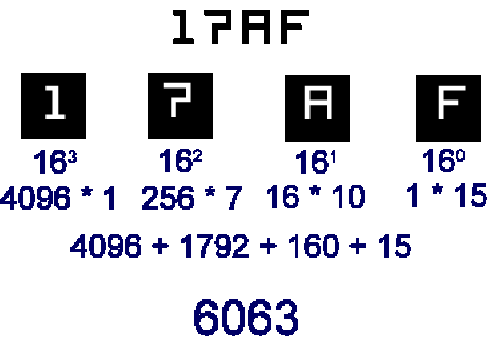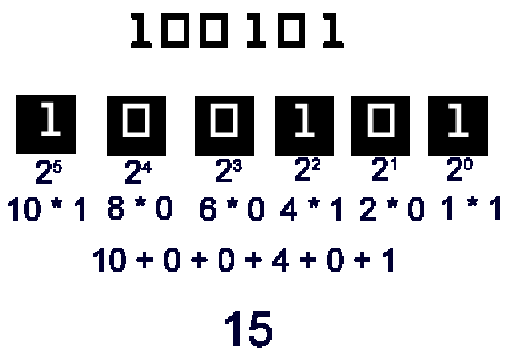# Number Systems for Dummies

Why Learn The Number Systems?

When humans are speaking to one another, they speak in a particular language. This language is made of words and letters.

Although we type words and letters in the computer, the computer does not understand the words and letters. Rather, those words and letters are translated into numbers.

Computers “talk” and understand in numbers. As a computer programmer or an IT professional, you need to understand the three most common number systems that are frequently used in computers.

Those number systems are:

1. Decimal
3. Binary

If you have an understanding of the number systems, you will understand the following topics in computer science:

• You will understand how to calculate network addresses
• You will understand how to read memory address locations in core dumps
• You will understand the color definitions in web and application programming
• You will understand many other concepts

### The Decimal Number System

The decimal number system is the system that is commonly known with people because it is most frequently used in arithmetic and in everyday life.

The decimal number system is also known as the base-10 number system, because each position in the number represents an incremental number with a base of 10.

For example, the first position (the furthest right) is represented as 100.

The second position (one from furthest right) is represented as 101, and so forth.

To determine what the actual number is in each position, take the number that appears in the position, and multiply it by 10x, where x is the power representation.

For example, if a number appears in the furthest right position, take the number in the furthest right position and multiply it by 100.

If there are multiple positions in the number (ex: 1374), add all the results together.

Since the number system is represented in “tens”, there are only 10 numbers that can be a value in each position of the base-10 number. Each position can only contain a number between 0 and 9.

Let’s take a look at an example of interpreting a base-10 number. An example number in the decimal system is 1374. We know this number as “one thousand, three hundred and seventy four”. This is how to interpret the decimal number:The number 1374 is actually a representation of decimal positions. The first position at the furthest right of the number represents 100.

The next position represents 101.

The next position represents 102.

As we move further left of the number, each power is incremented by 1.

In order to determine what the number actually is in the position, take the number that appears in the position, and multiply it by 10x, where x represents the power.

Add all the numbers together from each position to find out what the number is.

The hexadecimal number system is used as an intermediary system in computers, such as a representation of memory addresses or a representation of colors.

The hexadecimal number system is also known as the base-16 number system, because each position in the number represents an incremental number with a base of 16.

For example, the first position (the furthest right) is represented as 160. The second position (one from furthest right) is represented as 161, and so forth.

To determine what the actual number is in “decimal” representation, take the number that appears in the position, and multiply it by 16x, where x is the power representation.

For example, if a number appears in the furthest right position, take the number in the furthest right position and multiply it by 160. If there are multiple positions in the number (ex: 17AF), add all the results together.

Since the number system is represented in “sixteens”, there are only 10 numbers and 5 letters that can be a value in each position of the base-16 number. Below are the numbers that each position can hold:

 Hexadecimal Representation “Decimal” Value 0 0 1 1 2 2 3 3 4 4 5 5 6 6 7 7 8 8 9 9 A 10 B 11 C 12 D 13 E 14 F 15

Let’s take a look at an example of interpreting a base-16 number. An example number in the hexadecimal format is 17AF. This is how to “interpret” the number to a decimal format:The number 17AF is a hexadecimal number. The first position at the furthest right of the number represents 160.

The next position represents 161.

The next position represents 162.

As we move further left of the number, each power is incremented by 1.

In order to determine what the number actually is in the position, take the number that appears in the position, and multiply it by 16x, where x represents the power.

Note that in the hexadecimal number system, letters A through F represent numbers 10 through 15, respectively.

Add all the numbers together from each position to find out what the decimal number is.

### The Binary Number System

The binary number system is the most basic number system that most machines (and electrical devices) use to communicate. The binary number system is also known as the base-2 number system, because each position in the number represents an incremental number with a base of 2.

For example, the first position (the furthest right) is represented as 20.

The second position (one from furthest right) is represented as 21, and so forth. To determine what the actual number is in each position, take the number that appears in the position, and multiply it by 2x, where x is the
power representation.

For example, if a number appears in the furthest right position, take the number in the furthest right position and multiply it by 20.

If there are multiple positions in the number (ex: 10011), add all the results together.

Since the number system is represented in “twos”, there are only 2 numbers that can have a value in each position of the base-2 number. Each position can only contain a 0 or a 1.

Let’s take a look at an example of interpreting a base-2 number. An example number in the decimal system is 100101. This is how to “interpret” the number in a decimal format:The number 100101 is a binary number. The first position at the furthest right of the number represents 20.

The next position represents 21.

The next position represents 22.

As we move further left of the number, each power is incremented by 1.

In order to determine what the number actually is in the position, take the number that appears in the position, and multiply it by 2x, where x represents the power.

Note that in the binary number system, the only two numbers that can appear in each position is either 0 or 1.

Add all the numbers together from each position to find out what the decimal number is.

error: Content is protected !!